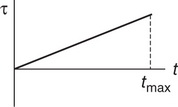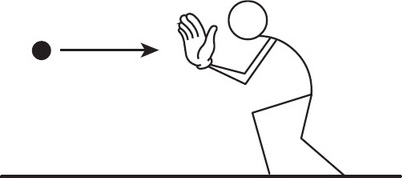# AP Physics 1 Multiple-select Practice Test 3

### Test Information9 questions18 minutes

1. The resistance of a sample of circular cross-section wire is known. A measurement of which two of the following would allow for a calculation of the resistivity of the wire? Select two answers.

2.The net torque τ on an object of rotational inertia I is shown as a function of time t. At time tmax, the object has speed ω and angular acceleration α. Which of the following methods correctly determines the change in the object’s angular momentum? Select two answers.

3. An object that's moving with constant speed travels once around a circular path. Which of the following statements are true concerning this motion? Select two answers.

4. Which of the following statements are true about uniformly accelerated motion? Select two answers.

5. An object moves at constant speed in a circular path. Which of the following statements are true? Select two answers.

6. Which of the following statements are true for a satellite in outer space orbiting the Earth in uniform circular motion? Select two answers.

7. Which of the following are characteristics of simple harmonic motion? Select two answers.

8. Which two velocity-versus-time graphs depict situations of uniformly accelerated motion? Select two answers.9. A man is standing on a frictionless surface. A ball is thrown horizontally to him, and he catches it with his outstretched hand, as shown above. Which two of the following values will remain the same after the catch as they were before the catch? Select two answers.